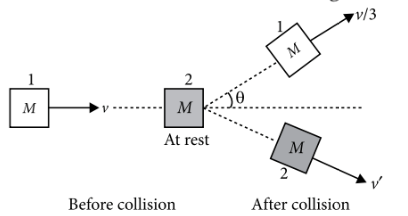Line of impact
Question

# On a frictionless surface, a block of mass M moving at speed $\nu$ collides elastically with another block of same mass M which is initially at rest. After collision the first block moves at an angle $\theta$ to its initial direction and has a speed $\frac{\nu }{3}$. The second block's speed after the collision is

Moderate
Solution

## The situation is shown in the figure.Let $\nu \text{'}$ be speed of second block after the collision. As the collision is elastic, so kinetic energy is conserved. According to conservation of kinetic energy,$\frac{1}{2}M{v}^{2}+0=\frac{1}{2}M{\left(\frac{v}{3}\right)}^{2}+\frac{1}{2}M{v}^{\text{'}2}$${v}^{2}=\frac{{v}^{2}}{9}+{v}^{\text{'}2}$${v}^{\text{'}}=\sqrt{\frac{8}{9}{v}^{2}}=\frac{\sqrt{8}}{3}v=\frac{2\sqrt{2}}{3}v$

Get Instant Solutions